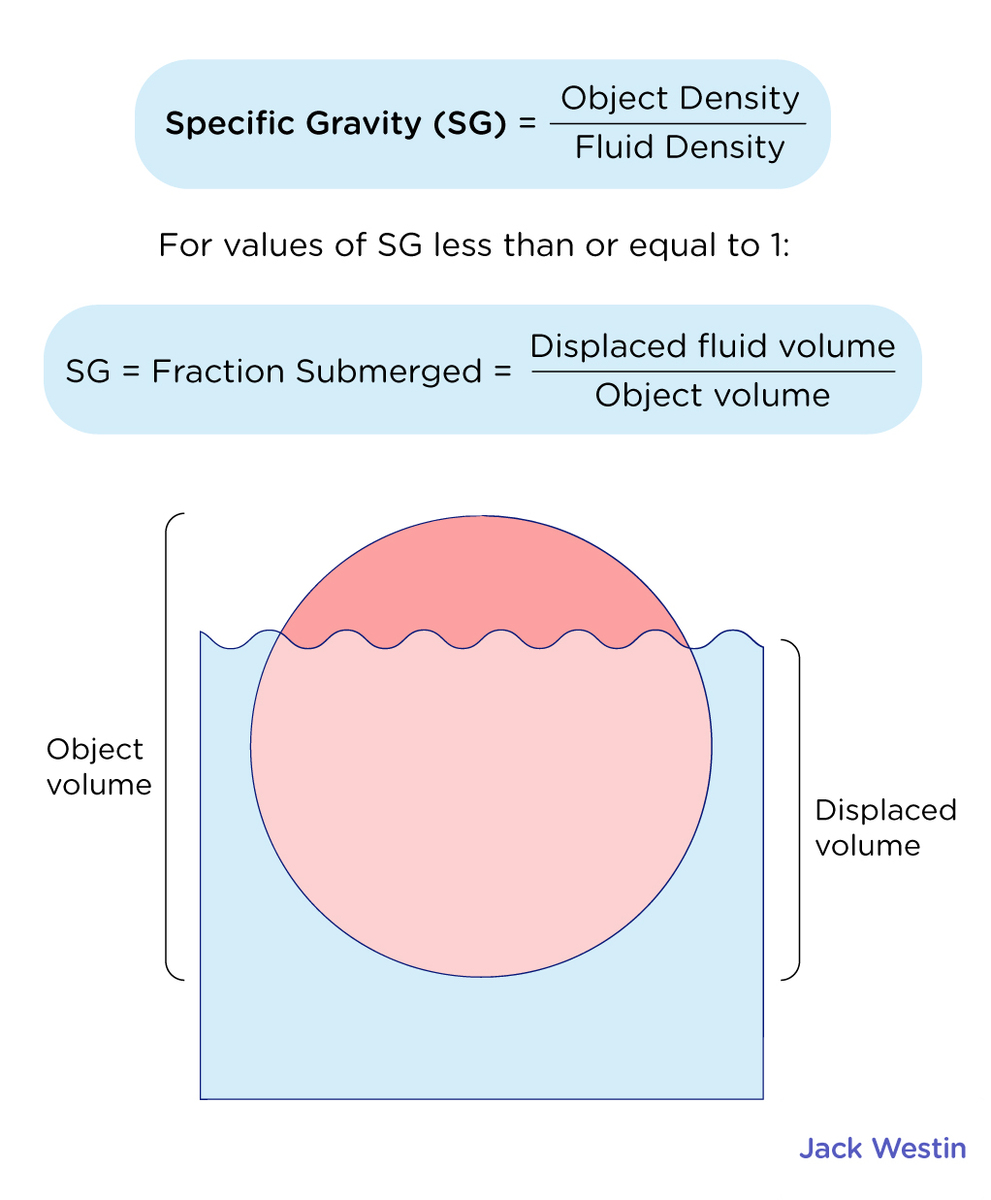Cyber Sale - Only 3 Days Remaining: ALL MCAT Products & Admissions Services

MCAT Content / Fluids / Density Specific Gravity

### Density, Specific Gravity

Topic: Fluids

Density refers to the amount of mass per unit of volume of an object while specific gravity is defined as the ratio of the density of the material to the density of water at 4.0ºC and one atmosphere of pressure (1000 kg/m3).

Liquids and gases are considered to be fluids because they yield to shearing forces, whereas solids resist them. In this article, gases and liquids will be referred to as fluids, making a distinction between them only when they behave differently.

Density is an important characteristic of substances. It refers to the amount of mass per unit of volume of an object. It is crucial, for example, in determining whether an object sinks or floats in a fluid. The average density of a substance or object is defined as its mass per unit volume:

where p (rho) is the symbol for density, m is the mass, and V is the volume. The SI unit of density is The metric system was originally devised so that water would have a density of 1g/cm3, equivalent to 103kg/m3.

The density of an object may help identify its composition. The densities of liquids and solids are roughly comparable, consistent with the fact that their atoms are in close contact. The densities of gases are much less than those of liquids and solids, because the atoms in gases are separated by large amounts of empty space. The gases are displayed for a standard temperature of

and a standard pressure of 101.3 kPa, and there is a strong dependence of the densities on temperature and pressure.

The densities of the solids and liquids displayed are given for the standard temperature of

and the densities of solids and liquids depend on the temperature. The density of solids and liquids normally increases with decreasing temperature.

The density of a substance is not necessarily constant throughout the volume of a substance. If the density is constant throughout a substance, the substance is said to be a homogeneous substance. A solid iron bar is an example of a homogeneous substance. The density is constant throughout, and the density of any sample of the substance is the same as its average density. If the density of a substance were not constant, the substance is said to be a heterogeneous substance. A chunk of Swiss cheese is an example of a heterogeneous material containing both the solid cheese and gas-filled voids.

Density is a dimensional property; therefore, when comparing the densities of two substances, the units must be taken into consideration. For this reason, a more convenient, dimensionless quantity called the specific gravity is often used to compare densities. Specific gravity is defined as the ratio of the density of the material to the density of water at 4.0°C  and one atmosphere of pressure, which is 1000kg/m3:

Specific gravity=Density of material/Density of waterSpecific gravity, being dimensionless, provides a ready comparison among materials without having to worry about the unit of density. For instance, the density of aluminum is 2.7 in g/cm3, but its specific gravity is 2.7, regardless of the unit of density. Specific gravity is a particularly useful quantity with regard to buoyancy.

Practice Questions

A scale under water

Hydrotherapy and full body immersion

MCAT Official Prep (AAMC)

Physics Online Flashcards Question 4

Physics Online Flashcards Question 24

Chemistry Online Flashcards Question 11

Physics Question Pack Passage 12 Question 70

Physics Question Pack Question 91

Key Points

• Liquids and gases are considered to be fluids because they yield to shearing forces,

• Density is an important characteristic of substances. It refers to the amount of mass per unit of volume of an object.

• The average density of a substance or object is defined as its mass per unit volume, ρ = m/ V.

• Densities of gases are much less than those of solids and liquids.

• Specific gravity is the ratio of the density of the material to the density of water. Specific gravity is dimensionless and used to compare densities.

• The density of a substance is not necessarily constant throughout the volume of a substance. If the density is constant throughout a substance, the substance is said to be a homogeneous substance. If the density of a substance were not constant, the substance is said to be a heterogeneous substance.

Key Terms

Homogeneous substance: The density is constant throughout a substance.

Heterogeneous substance: The density of a substance is not constant.

Density: Mass per unit volume of a substance or object.

Fluids: Liquids and gases; a fluid is a state of matter that yields to shearing forces

Pressure: Force per unit area exerted perpendicular to the area over which the force acts.

Specific gravity: The ratio of the density of an object to a fluid (usually water).

Billing Information